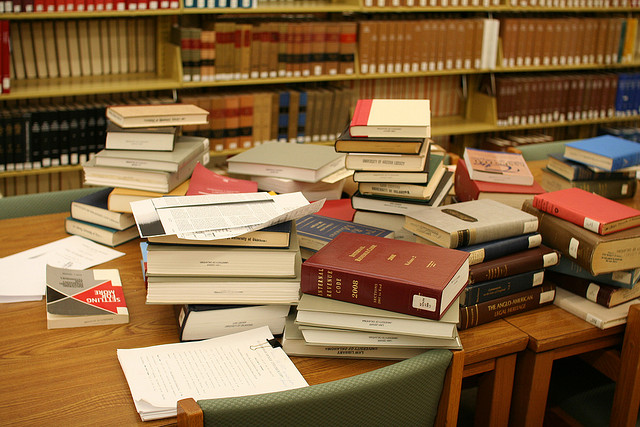# Math, Physics and Chemistry books

Probability Level 1On a table, there are a total of 30 distinct books: 9 math books, 10 physics books, and 11 chemistry books.

What is the probability of getting a book that is not a math book?

×